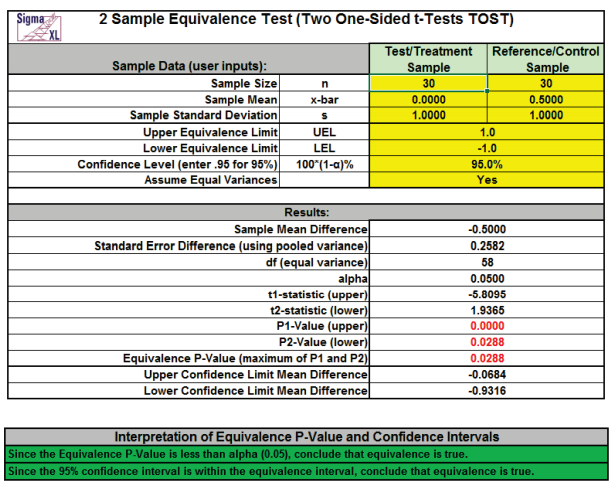Include Top

Basic Statistical Templates – 2 Sample Equivalence Test (Compare 2 Means)

Click SigmaXL > Templates & Calculators > Basic Statistical Templates > 2 Sample Equivalence Test (Compare 2 Means) to access the 2 Sample Equivalence Test (Two One-Sided t-Tests TOST) calculator. The template gives the following default example.Notes
1. Enter summarized Sample Data, Upper & Lower Equivalence Limits and Confidence Level in cells with yellow highlight. Do not modify any other part of this worksheet.
2. Select "Assume Equal Variances" using drop-down.
3. Null hypothesis for P1: Difference >= UEL; Null hypothesis for P2: Difference <= LEL. Both null hypotheses must be rejected to conclude that equivalence is true.
4. LEL and UEL establish the zone or region of equivalence and are determined by what size difference is considered practically significant.
5. This calculator will automatically detect the version of Excel and use the appropriate statistical functions.

# Web Demos

Our CTO and Co-Founder, John Noguera, regularly hosts free Web Demos featuring SigmaXL and DiscoverSim